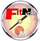# Row FixtureA RowFixture compares rows in the test data to objects in the system under test. Methods are invoked on the objects and returned values compared to those in the table. An algorithm matches rows with objects based on one or more keys. Objects may be missing or in surplus and are so noted.

See examples using row fixtures.

See source.

Q: RowFixture.match is kind of hard to understand. Obviously I did not know anything about it but some more info on its matching algorithm on its wiki page might help the novice reader like me. Do you think thatĀit could beĀrefactored to help the common brains like mine grok it?

A: RowFixture was very hard to get right until I found the current factoring. The algorithm is a variation on the bucket sort where two parallel sorts are taking place, one of the expected rows and another for the computed rows.

The fixture processes all the rows of one table following these five steps:

• bind the columns to variables and methods by reflection.
• query to get the result rows which will be checked.
• match the expected and result rows and check the matches.
• build html for missing rows.
• mark mark missing and surplus rows as such.

Of these steps the match is the most complex. The matching algorithm is one of divide and conquer using a method that calls itself recursively in two circumstances:

• recurse matching subsets of the expected and computed rows distinguished by unique values in a specific column.

• recurse so as to examine the next available column when the current column doesn't successfuly bind to any field or method.

Each invocation of the method divides the expected and computed rows into sublists with equal values in the current column of interest. (This is the "sort" step, as in sorting clothes into like piles.) Each sublist (pile) is considered in turn. There are four cases:

• the expected list is empty so computed rows are surplus.
• the computed list is empty so expected rows are missing.
• there is exactly one row in each list so compare them.
• otherwise the match is ambiguous so further sorting on subsequent columns is required.

The method uses several helper functions which are sometimes duplicated because of representational differences between the expected rows (Parse objects) and the computed rows (Domain objects).

See the perl spike of the matching algorithm.

Last edited July 17, 2003